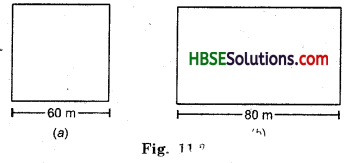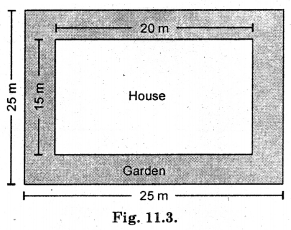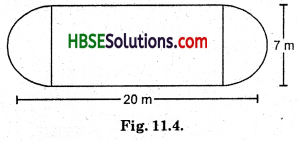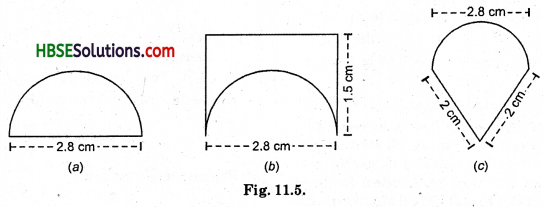# HBSE 8th Class Maths Solutions Chapter 11 Mensuration Ex 11.1

Haryana State Board HBSE 8th Class Maths Solutions Chapter 11 Mensuration Ex 11.1 Textbook Exercise Questions and Answers.

## Haryana Board 8th Class Maths Solutions Chapter 11 Mensuration Exercise 11.1

Question 1.
A square and a rectangular field with measurements as given in the figure have the same perimeter. Which field has a larger area ?Solution:
(a) Perimeter of square
= 4 × side
= 4 × 60 = 240 m

(b) Perimeter of rectangle = 2 (l + b)
240 m = 2(80 + b)
$$\frac{240}{2}$$ = 80 + b
120 = 80 + b
120 – 80 = b
40 = b
∴ b = 40 m
∴ Area of square = (side)2 = (60)2
60 × 60 = 3600 m2
and area of rectangle = l × b = 80 m × 40 m
= 3200 m2
Hence square field is larger than rectangle.Question 2.
Mrs. Kaushik has a square plot with the measurement as shown in the figure. She wants to construct a house in the middle of the plot. A garden is developed around the house. Find the total cost of developing a garden around the house at the rate of Rs. 55 per m2.Solution:
Area of garden
= Area of square plot – Area of middle plot (Rectangular plot)
= (side)2 – (l × b)
= (25)2 – (20 × 15)
= 625 – 300 = 325 m2
Hence the total cost of developing garden
= 325 m2 × Rs. 55 = Rs. 17875.

Question 3.
The shape of a garden is rectangular in the middle and semi-circular at the ends as shown in the diagram. Find the area and the perimeter of this garden (Length of rectangle is 20 – (3.5 + 3.5) metres].Solution:
Length of rectangle
= 20 – (3.5 + 3.5)
= 20 – 7 = 13 m
Breadth of rectangle = 7 m
Radius of semi-circle = 3.5 cm
Two semicircle = one circle
Hence perimeter (or circumference) of circle
= 2pr = 2 × $$\frac{22}{7}$$ × 3.5 = 22 cm
Perimeter of rectangle
= 2 (l + b)
= 2 × (13 + 7)
= 2 × 20 = 40 cm
Hence, perimeter of this garden
=(22 cm + 40 cm) = 62 cm
Now, area of rectangle
= l × b = 13 × 7 = 91 cm2
Area of circle (two semicircle)
= πr2 = $$\frac{22}{7}$$ × 3.5 × 3.5
= 38.5 cm2
Hence, area of garden
= 38.5 cm2 + 91 cm2
= 129.5 cm2.Question 4.
A flooring tile has the shape of a parallelogram whose base is 24 cm and the corresponding height is 10 cm. How many such tiles are required to cover a floor of area 1080 m2 ? (If required you can split the tiles in whatever way you want to fill up the corners).
Solution:
Area of parallelogram tiles
= base × height
= b × h
= 24 × 10 = 240 cm2
Area of parallelogram
= 1080 m2
= 1080 × 100 × 100 × cm2
= 10800000cm2
∴ Number of tiles
= $$\frac{Area of floor}{7}$$
= $$\frac{10800000}{240}$$ = 45000.Question 5.
An ant is moving around a few foodSolution:
(a) Circumference (perimeter) of semicircle
= $$\frac{2 \pi r}{2}$$ = $$\frac{2 \times 22 \times 1.4}{2 \times 7}$$
(d = 2.8 cm, r = 1.4 cm)
= 4.4 cm
Perimeter = 4.4 cm + 2.8 cm = 7.2 cm.

(b) Circumference of semicircle + Perimeter of square
= 4.4 cm + 1.5 cm + 2.8 cm + 1.5 cm
= 10.2 cm.

(c) Circumference of semicircle + Perimeter of triangle
= 4.4 cm + 2 cm + 2 cm
= 8.4 cm
Hence (b) food piece would the ant have to take a longer round.